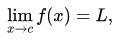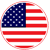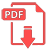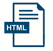# Home | Mathematics | Limits

## Determinate Limits

Cauchy and Heine Definitions of Limit

Let f(x) be a function that is defined on an open interval X containing x=a. (The value f(a) need not be defined.)

The number L is called the limit of function f(x) as x→a if and only if, for every ε>0 there exists δ>0 such that

|f(x)−L|<ε,

whenever

0<|x−a|<δ.

This definition is known as ε−δ− or Cauchy definition for limit.

There’s also the Heine definition of the limit of a function, which states that a function f(x) has a limit L at x=a, if for every sequence {xn}, which has a limit at a, the sequence f(xn) has a limit L. The Heine and Cauchy definitions of limit of a function are equivalent.

In formulas, a limit of a function is usually written asand is read as "the limit of f of x as x approaches c equals L". The fact that a function f approaches the limit L as x approaches c is sometimes denoted by a right arrow (→), as inEnglish